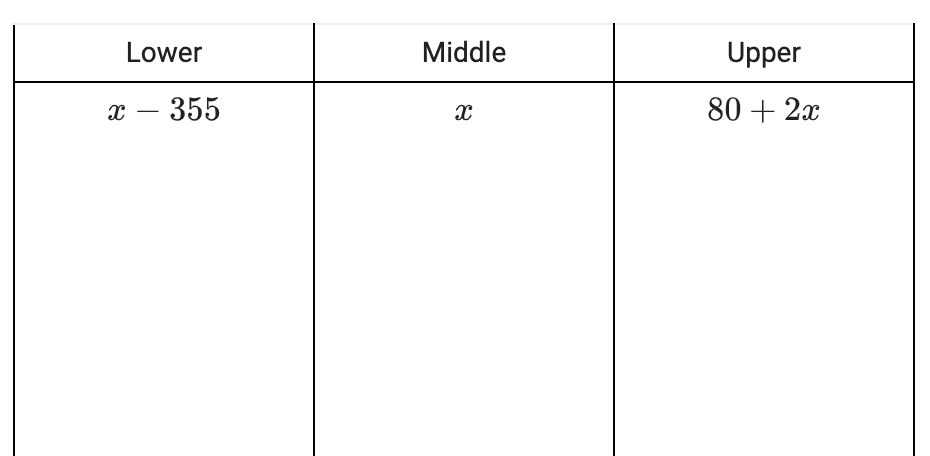### Home > CC2MN > Chapter 7 > Lesson 7.2.5 > Problem7-111

7-111.

Use the 5-D Process to solve the following problem. Write an expression to represent each column of your table.

Yosemite Falls, the highest waterfall in the United States, is actually made up of three smaller falls. The Lower Yosemite Falls is $355$ feet shorter than the Middle Cascades Falls. The Upper Yosemite Falls is $80$ feet more than twice the Middle Cascades Falls. If the entire set of waterfalls is $2425$ feet long, how tall is each of the smaller waterfalls?

Write an expression for the height of each falls. 3 column table, first row labeled as follows: Lower, middle, upper,  Row 2 labeled as follows, first: x minus 355, Second: x, Third: 80 + 2 x,Now note that the sum of these expressions is $2425$ feet.

$(x-355)+(x)+(80+2x)=2425$
Solve for $x$.

Remember that $x=\text{the height of the middle falls}$.
Use the value you found for $x$ to calculate the height of the lower and upper falls.